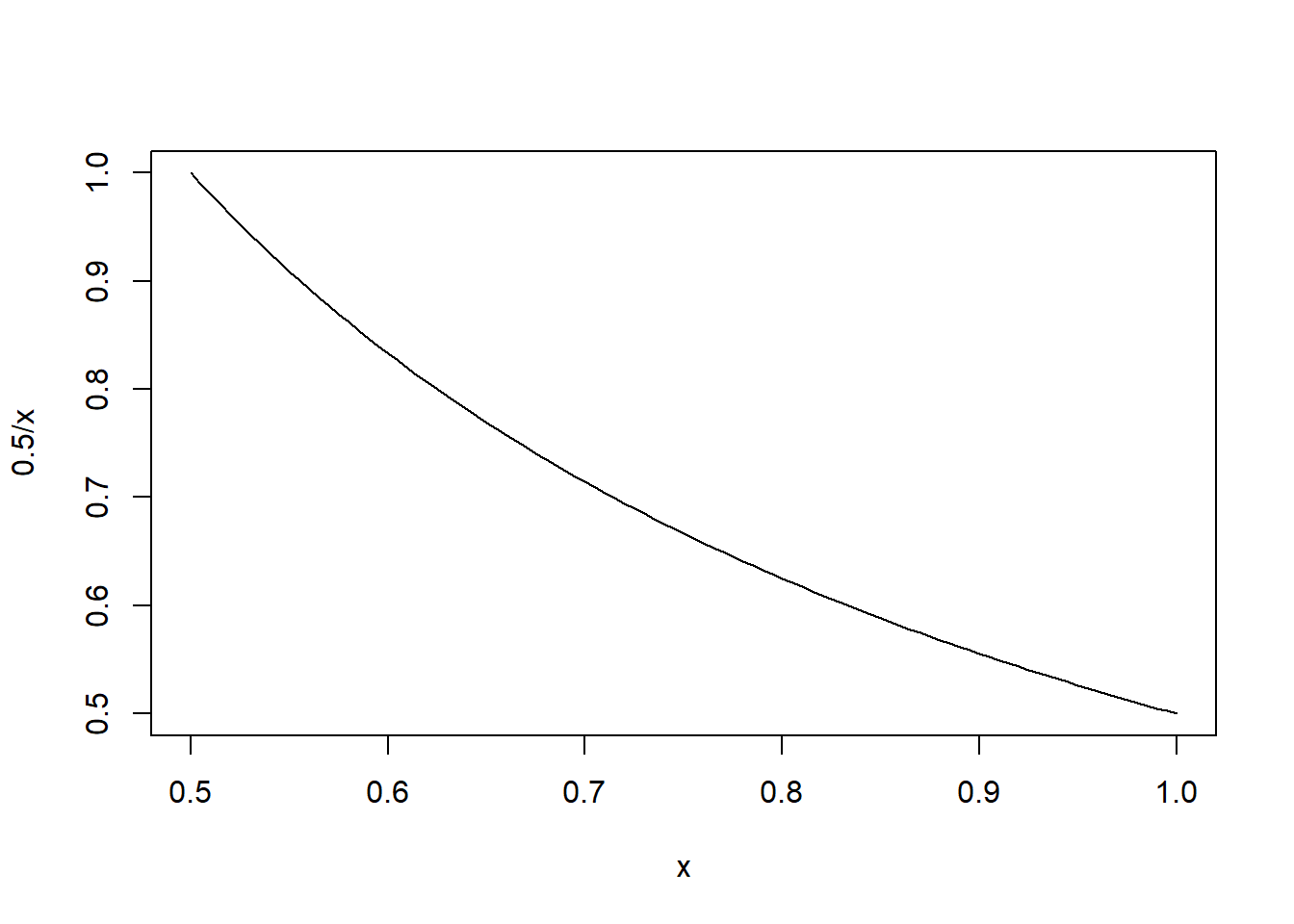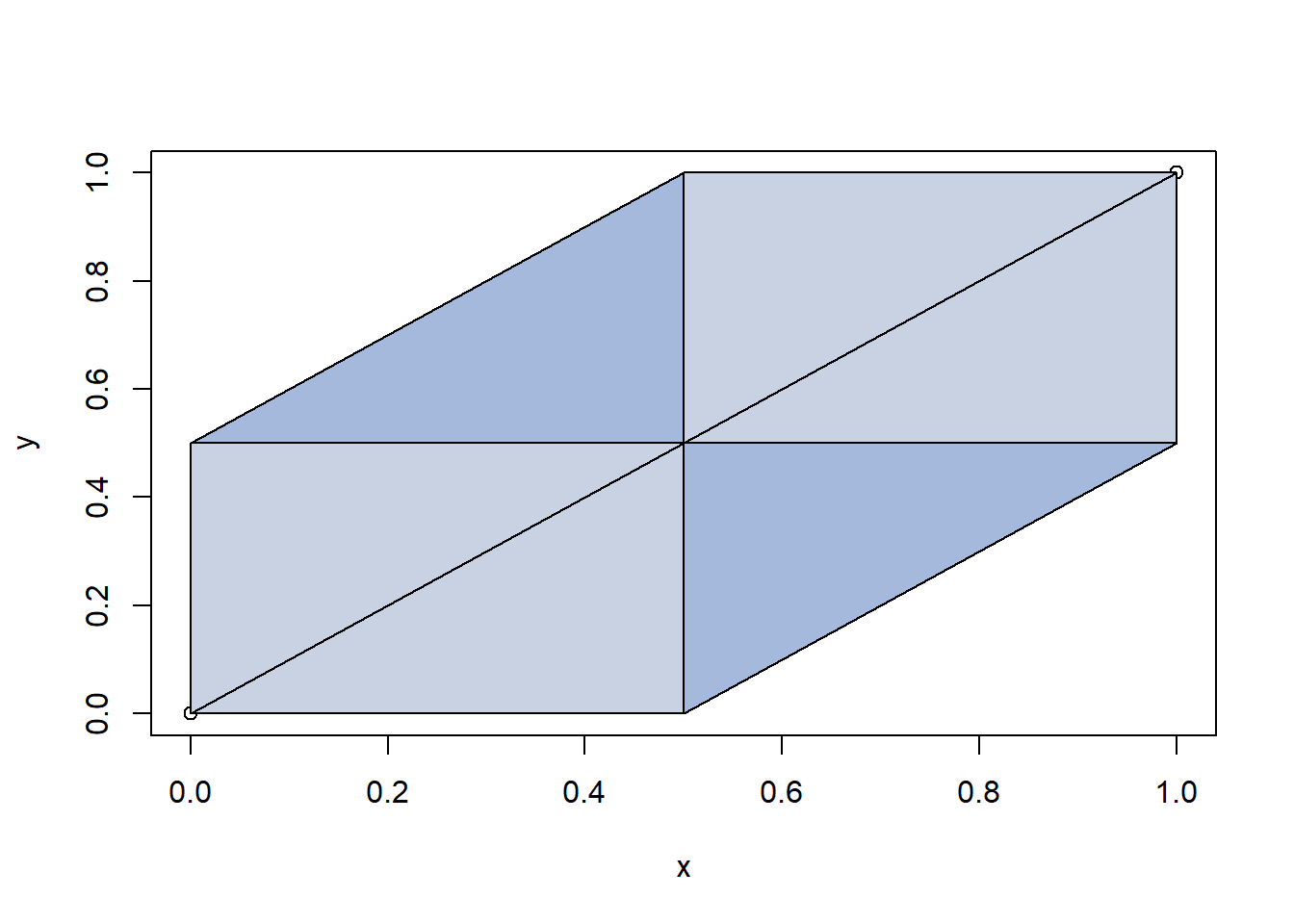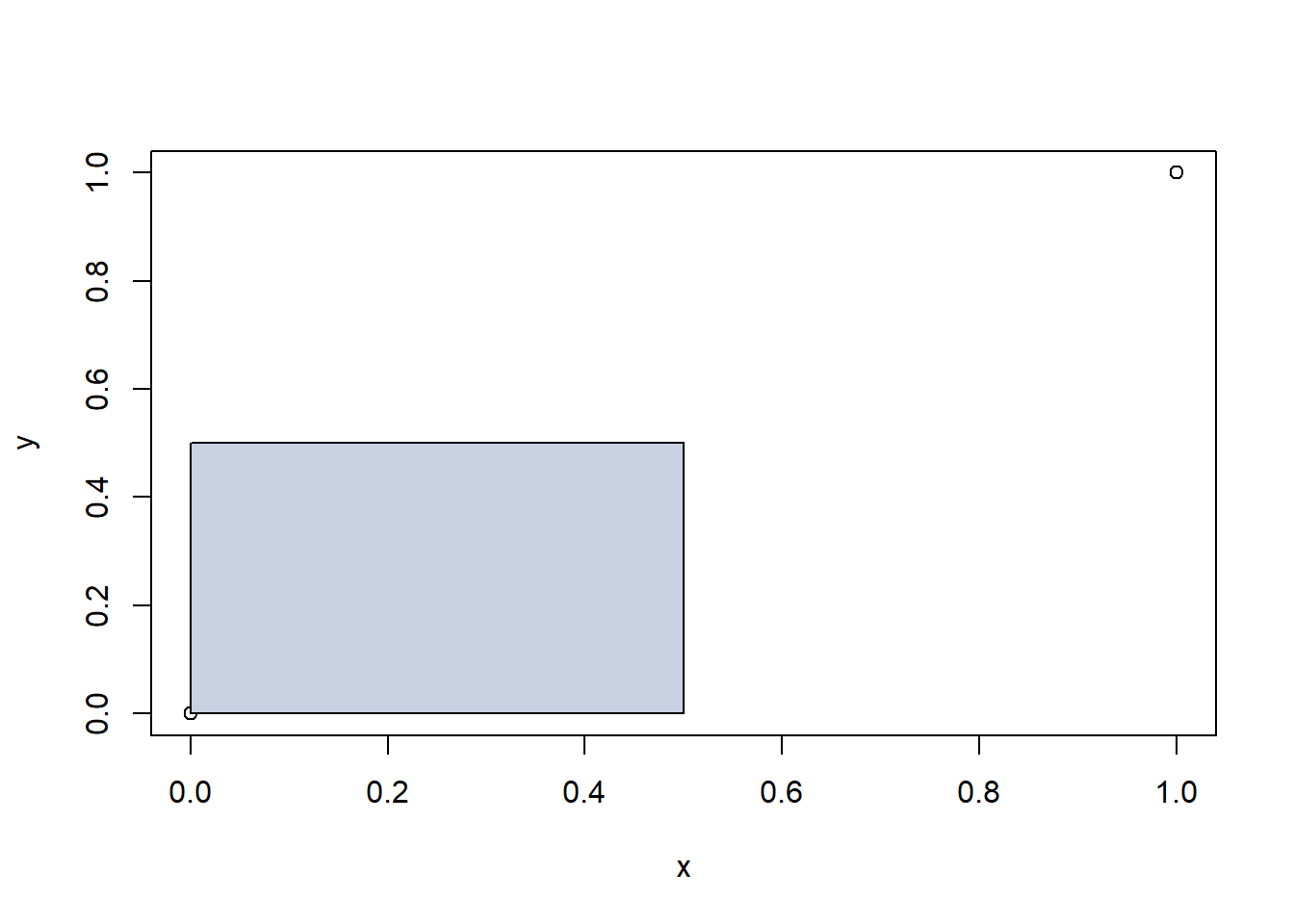# Probability Distributions

Choose independently two numbers B and C at random from the interval [0, 1] with uniform density. Prove that B and C are proper probability distributions. Note that the point (B,C) is then chosen at random in the unit square.

A proper probability function has probabilities between 0 and 1 and the sum of the probabilities of the outcomes must be 1. B and C both represent probabilities between 0 and 1 and the probability of each event occuring adds up to 1.

Find the following probabilities:

## Part 1: $B + C < \frac{1}{2}$

### Theoretical Solution

To find the probability, set up the joint cumulative distribution integrals to find the area of b + c < 1/2 on the unit square.

$\int_0^{\frac{1}{2}}\int_0^{\frac{1}{2}} (b + c)~db~dc$ $\int_0^{\frac{1}{2}}[\frac{1}{2}b^2 + bc]^{\frac{1}{2}}_{0}~dc$

$\int_0^{\frac{1}{2}}(\frac{1}{8} + \frac{1}{2}c)~dc$ $[\frac{1}{8}c + \frac{1}{4}c^2]^{\frac{1}{2}}_{0}$

$(\frac{1}{16} + \frac{1}{16})$

$P(B + C < \frac{1}{2}) = \frac{1}{8}$

### Experimental Solution

This can be confirmed with the following trial. Because of the Law of Large Numbers, we can run a simulation to test the experimental probability of this event.

count = 0
trials = 100000
for (i in (1:trials)){
B = runif(1, min=0, max=1)
C = runif(1, min=0, max=1)
if(B + C < 0.5){
count = count + 1
}
}
print(count/trials)
##  0.1248

## Part 2: $BC < \frac{1}{2}$

### Theoretical Solution

For this example, we have to find the area under the following curve:

$bc = \frac{1}{2} => c = \frac{1}{2b}$ This curve is inf at x = 0 , so instead we will only find the area under the curve from x = [0.5, 1]. At x = 0.5, y = 1. This tells us that the area between x = [0, 0.5] and y = [0, 1] is 0.5.

curve(.5/x, from = 0.5, to = 1)To find the area between x = [0.5, 1] and y = [0, 1], we set up an the following integral.

$\int_0^{1}\int_\frac{1}{2}^{1} \frac{1}{2b}~db~dc$

$\int_0^{1}[\frac{ln(2b)}{2}]^1_{\frac{1}{2}}~dc$

$\int_0^{1}0.346573~dc = 0.346573$

$P(BC < \frac{1}{2}) = \frac{1}{2} + ~0.346573 = ~0.846573$

### Experimental Solution

Confirming this approximate result with the experimental value:

count = 0
trials = 10000
for (i in (1:trials)){
B = runif(1, min=0, max=1)
C = runif(1, min=0, max=1)
if(B * C < 0.5){
count = count + 1
}
}
print(count/trials)
##  0.848

## Part 3: $|B - C| < \frac{1}{2}$

### Theoretical Solution

For this example, we have to find the area of the following components of the unit square:

x <- 0:1
y <- x
plot(x, y)

polygon(cbind(c(.5, x, 1), c(0, y, .5)), col="#a4b9db")
polygon(cbind(c(0, x, .5), c(.5, y, 1)), col="#a4b9db")
polygon(rbind(c(0,0),c(0,0.5),c(0.5,0.5),c(0.5,0)), col="#c8d2e3")
polygon(rbind(c(0.5,0.5),c(1,0.5),c(1,1),c(0.5,1)), col="#c8d2e3")
lines(x, y)The probability of these components of the unit square is:

$P(|B - C| < \frac{1}{2}) = \frac{3}{4}$

### Experimental Solution

Confirming this solution with an experimental value:

count = 0
trials = 10000
for (i in (1:trials)){
B = runif(1, min=0, max=1)
C = runif(1, min=0, max=1)
if(abs(B - C) < 0.5){
count = count + 1
}
}
print(count/trials)
##  0.7443

## Part 4: $max(B,C) < \frac{1}{2}$

### Theoretical Solution

The for the maximum of B and C to be less than 0.5, both B and C must be less than 0.5.

This represents the following portion of the unit square.

x <- 0:1
y <- 0:1
plot(x, y)

polygon(rbind(c(0,0),c(0,0.5),c(0.5,0.5),c(0.5,0)), col="#c8d2e3")Or, it can also be represented by the following probability (since A and B are independent).

$P(A < 0.5 \cup B < 0.5) = \frac{1}{2} * \frac{1}{2} = \frac{1}{4}$

### Experimental Solution

Confirming the theoretical solution with the experimental solution:

count = 0
trials = 100000
for (i in (1:trials)){
B = runif(1, min=0, max=1)
C = runif(1, min=0, max=1)
if(max(B, C) < 0.5){
count = count + 1
}
}
print(count/trials)
##  0.25058